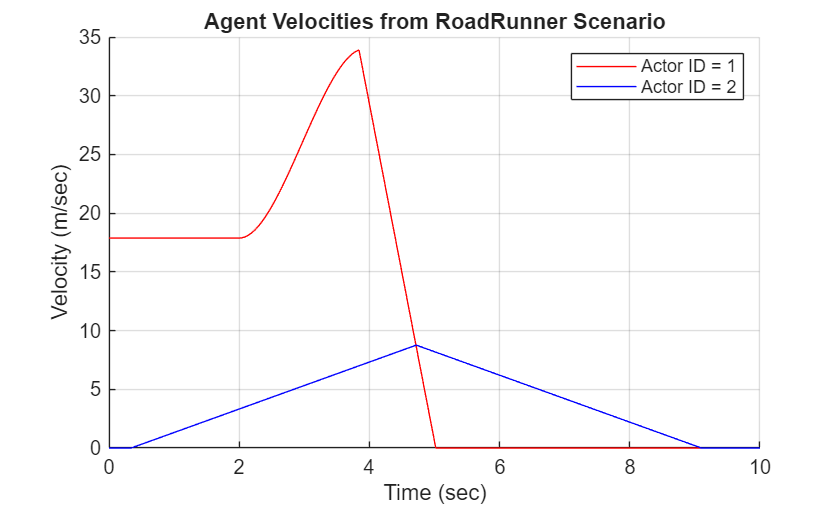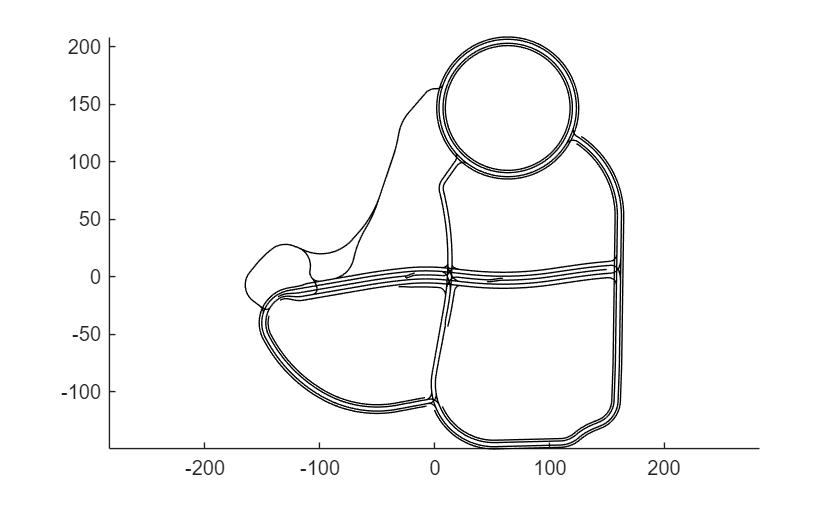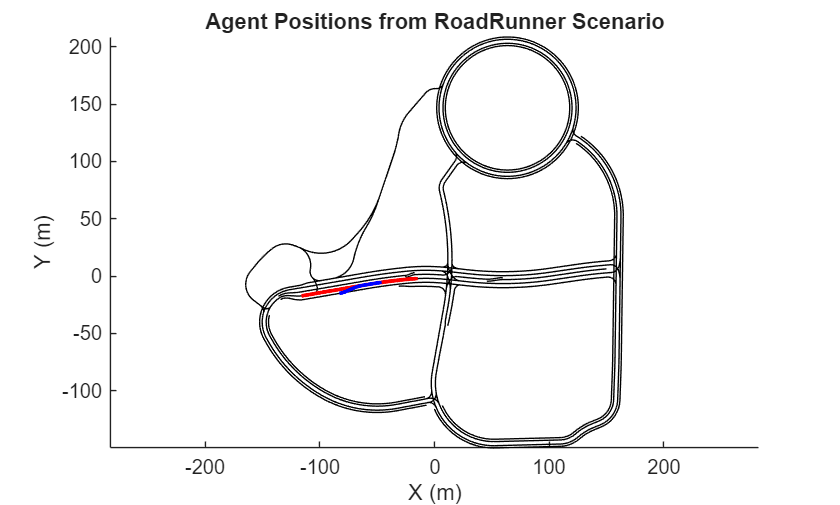# Simulate a RoadRunner Scenario Using MATLAB Functions

This example shows how to run and visualize scenarios in RoadRunner Scenario using MATLAB functions. You can use MATLAB functions to control RoadRunner Scenario programmatically. Common programmatic tasks that you can perform include:

• Open and close the RoadRunner Scenario application.

• Open, close, and save scenes, scenarios, and projects.

• Import and export scenarios.

RoadRunner Scenario enables you to interactively design and simulate agents in scenarios. To verify the behavior of these agents, it is often helpful to automate the process of running and analyzing the results of scenario simulations. In this example, you learn how to use Automated Driving Toolbox® to launch RoadRunner Scenario, configure and run a simulation, and then plot simulation results.

To run this example, you must:

• Have an Automated Driving Toolbox® license.

• Have created a RoadRunner project folder.

### Set Up Environment to Launch RoadRunner Scenario

To use MATLAB functions to control RoadRunner Scenario programmatically, use the `roadrunner` object. By default, `roadrunner` opens RoadRunner from the default installation folder for the platform you are using (either Windows® or Linux®). These are the default installation locations by platform:

• Windows – C:\Program Files\RoadRunner R20NNx\bin\win64

`R20NNx` is the MATLAB release you are using.

If your RoadRunner Scenario installation is at a different location than the default location, use the MATLAB `settings` API to customize the default value of the RoadRunner Scenario installation folder.

You can use the `roadrunner` function to create a `roadrunner` object and launch a RoadRunner Scenario session. The `roadrunner` function requires an argument that specifies the location of a RoadRunner project. A RoadRunner project folder typically contains these subfolders: `Assets`, `Exports`, `Project`, `Scenarios`, and `Scenes`.

Open a project in RoadRunner using the `roadrunner` function by specifying the location in which to create a project. This example assumes that RoadRunner is installed in its default location in Windows.

Specify the path to an existing project. For example, this code shows the path to a project located on `C:\RR\MyProject`. The function returns a `roadrunner` object, `rrApp,` that provides functions for performing basic workflow tasks such as opening, closing, and saving scenes and projects.

```rrProj = "C:\RR\MyProject"; rrApp = roadrunner(rrProj,InstallationFolder="C:\Program Files\RoadRunner R2022b\bin\win64");```

Open an existing scenario in RoadRunner Scenario by using the `openScenario` function and specifying the `rrApp` object and the specific scenario `filename` that you want to open. For example, open the `TrajectoryCutIn` scenario file, which is a scenario included by default in RoadRunner projects. This function opens the desired scenario in the RoadRunner Scenario application through MATLAB.

`openScenario(rrApp,"TrajectoryCutIn.rrscenario");`

### Simulate Scenario

Once the scenario is loaded into RoadRunner Scenario, automate the simulation tasks by using the `createSimulation` function to create a simulation object. The simulation object enables you to programatically interact with the scenario simulation.

Specify the `rrApp` object as an input argument to the `createSimulation` function. The function creates a simulation object, `rrSim`.

`rrSim = createSimulation(rrApp);`
```Connection status: 1 Connected to RoadRunner Scenario server on localhost:59151, with client id {1c6fd5fc-736d-4cd9-9d60-84bc96444b95} ```

Set a maximum simulation time of 10 seconds. Use the `set` function and specify the `rrSim` object, name of the variable to set, and the value for that variable as input arguments.

```maxSimulationTimeSec = 10; set(rrSim,'MaxSimulationTime',maxSimulationTimeSec);```

Enable simulation result logging so that you can plot the results later.

`set(rrSim,"Logging","on");`

Start the simulation. Use a `while` loop to monitor the status of the simulation, and wait for the simulation to complete.

```set(rrSim,"SimulationCommand","Start"); while strcmp(get(rrSim,"SimulationStatus"),"Running") pause(1); end```

### Plot Agent Velocities

In this section, you retrieve the logged velocities of the actors from the simulation and plot their magnitudes against simulation time.

Get the logged results from the scenario. Use the `get` function and specify the `rrSim` object and "`SimulationLog"` as input arguments. The function returns the simulation logs in `rrLog`, which contains information about the simulation of the scenario.

`rrLog = get(rrSim,"SimulationLog");`

The `TrajectoryCutIn scenario` contains two actors. The red sedan has `Actor ID` set to `1,` and the white sedan has `Actor ID` set to `2`. Get the logged velocities of these actors from simulation log. Also, get the corresponding simulation times from the simulation logs.

```velocityAgent1 = get(rrLog,'Velocity','ActorID',1); velocityAgent2 = get(rrLog,'Velocity','ActorID',2); time = [velocityAgent1.Time];```

The function returns the velocities of the red sedan and the white sedan as vectors and stores them in the `velMagAgent1` and `velMagAgent2` variables, respectively. Calculate the magnitude of the velocity for each actor by using the `norm` function.

```velMagAgent1 = arrayfun(@(x) norm(x.Velocity,2),velocityAgent1); velMagAgent2 = arrayfun(@(x) norm(x.Velocity,2),velocityAgent2);```

Plot the agent velocities with respect to simulation time using the `plot` function. Label the graph and the x and y axes.

```figure hold on plot(time,velMagAgent1,"r") plot(time,velMagAgent2,"b") grid on title("Agent Velocities from RoadRunner Scenario") ylabel("Velocity (m/sec)") xlabel("Time (sec)") legend("Actor ID = 1","Actor ID = 2")```Notice that the velocities of the actors correspond to their specifications in the Logic Editor of RoadRunner Scenario.

### Plot Agent Velocities

Plot the lanes from the RoadRunner scene and overlay the positions of vehicles on the map.

Get the HD Map specification from RoadRunner by using the `getMap` function. Notice that the function returns a structure and one of the fields contains information about the lanes.

```hdMap = getMap(rrSim); lanes = hdMap.map.lanes;```

Loop through each of the lane specifications using a `for` loop and plot the lane coordinates.

```figure hold on for i = 1:numel(lanes) control_points = lanes(i).geometry.values; x_coordinates = arrayfun(@(cp) cp.x,control_points); y_coordinates = arrayfun(@(cp) cp.y,control_points); plot(x_coordinates, y_coordinates, 'black'); end axis equal```Extract the positions of the vehicles and plot them on the lanes.

```poseActor1 = rrLog.get('Pose','ActorID',1); positionActor1_x = arrayfun(@(x) x.Pose(1,4),poseActor1); positionActor1_y = arrayfun(@(x) x.Pose(2,4),poseActor1); plot(positionActor1_x,positionActor1_y,"r","LineWidth",2) poseActor2 = rrLog.get('Pose','ActorID',2); positionActor2_x = arrayfun(@(x) x.Pose(1,4),poseActor2); positionActor2_y = arrayfun(@(x) x.Pose(2,4),poseActor2); plot(positionActor2_x,positionActor2_y,"b","LineWidth",2) title("Agent Positions from RoadRunner Scenario") ylabel("Y (m)") xlabel("X (m)")````figure(gcf)`

### Close Scenario Session

To stop interacting with RoadRunner Scenario, close the simulation. Then close the application.

`close(rrApp)`

Close the open figure.

`close all`

### Further Exploration

In this example you learned about the basic capabilities of connecting to RoadRunner Scenario programmatically using MATLAB. To extend this script further, you can:

• Vary the scenario and vehicle actors in the scenario.

• Develop MATLAB and Simulink behaviors, publish actor behavior, simulate behaviors in RoadRunner Scenario simulation, and control simulations and access simulation parameters.